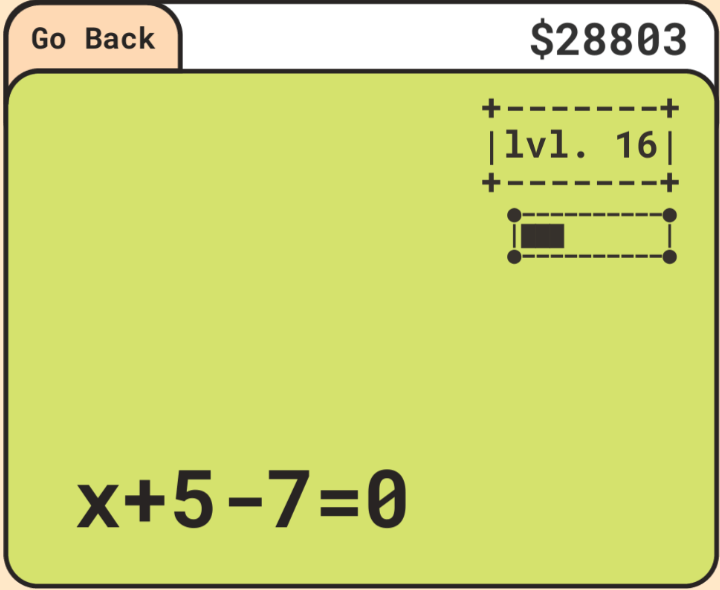## Optimizing The Code

To start this off I changed my variables from int1 to a to be more readable and less-bug prone.

``````// OLD VERSION
// x+a=b => x=b-a
ans = int2-int1;
prompt.text = \$"x+{int1}={int2}";
// NEW VERSION
// x+a=b => x=b-a
x = b-a;
prompt.text = \$"x+{a}={b}";``````

The new formating to use the alphabet a,b,c makes the comments seamlessly transition into the code itself.

REFINING PROBLEM GENERATION – incorrect probabilities

``````// some code was delete for simplicity of demonstration
if (UnityEngine.Random.Range(0,101) > 50){
if(UnityEngine.Random.Range(0,101) > 67){
if(UnityEngine.Random.Range(0,101)>50){
if(UnityEngine.Random.Range(0,101)>50){``````

Each one of these if statements would have to calculate a new probability. This first causes an issue of unneeded processing time as you could have the percent generate once at the beginning. Another issue is for a problem that uses something like:

``````if(UnityEngine.Random.Range(0,101) > 66) {}
else if (UnityEngine.Random.Range(0,101) > 33) {}
else {}``````

The issue with this code is that if the intial if statment generated a probability of 50 then it would go to the else if. Ideally the else if would execute since its between 66 and 33.

``````CHANCE OF GETTING THE SECOND OPTION
(66-33)/100 = .33 AKA 33%

VS.

(66-0)/100 AND (100-33)/100 =  0.4422 AKA 44%``````

This means with the current code you have a 44% of getting the second option and 22% of getting the last option when they all should be 33%. To remedy this all of the if statements will use the same original probability.

``````int randomPercent = (int) UnityEngine.Random.Range(0, 101);
if(randomPercent > 66) {}
else if (randomPercent > 33) {}
else {}``````

However; once inside an if statement I will use a new randomPercent variable to have the events be mutually exclusive.

ABSTRACTION – fewer lines

I justified adding a new function as in the long run it should cut every 2 lines of code into just 1 line. I think it should scale as well.

``````// OLD CODE
prompt.text = \$"x+{a}={c}+{b}";
Debug.Log("Algebra Problem ID: 5");

// NEW CODE
displayPrompt(\$"x+{a}={c}+{b}", 6);

// Function
void displayPrompt(String displayPrompt, int problemID)
{
Text prompt = problemPrompt.GetComponent<Text>();
prompt.text = displayPrompt;
Debug.Log(\$"Algebra Problem ID: {problemID}");
}``````

BUGS WHILE CODING

1. Incorrect displaying. While copying and pasting I copy and pasted the incorrect prompt so I was getting the “wrong answer”
2. Same problem. Because of the percent changes I was getting the same percent over and over again because of scoping issues.

## Closing Notes

I have now completed the subtraction problems for the Algebra Archive stage. If I hadn’t mentioned this in the last post I also have the problems broken into tiers. The first tier is for only problems like x+a=b while the second tier implements a third variable, x+a+b=c.